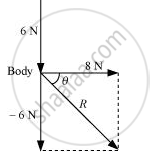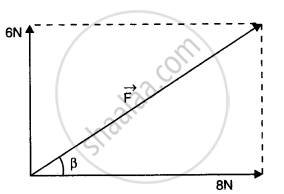# A Body of Mass 5 Kg is Acted Upon by Two Perpendicular Forces 8 N and 6 N. Give the Magnitude and Direction of the Acceleration of the Body - Physics

A body of mass 5 kg is acted upon by two perpendicular forces 8 N and 6 N. Give the magnitude and direction of the acceleration of the body

#### Solution 1

2 m/s2, at an angle of 37° with a force of 8 N

Mass of the body, m = 5 kg

The given situation can be represented as follows:The resultant of two forces is given as:

R = sqrt((8)^2+(-6)^2) = sqrt(64+36) = 10N

θ is the angle made by R with the force of 8 N

:. theta = tan^(-1) ((-6)/8) = -36.87^@

The negative sign indicates that θ is in the clockwise direction with respect to the force of magnitude 8 N.

As per Newton’s second law of motion, the acceleration (a) of the body is given as:

ma

:. a = F/m = 10/5 = 2 "m/s"^2

#### Solution 2Here m = 5 kg

F_1 = 8 N and F_2 = 6 N

The resultant force on the body

F =sqrt(F_1^2 + F_2^2) = sqrt(8^2 + 6^2 N)

=> F= sqrt(64 + 36)N = 10 N

The acceleration a  = F/m

=> a = 10/5  = 2 ms^(-2) in the same direction as the resultant force

The direction of acceleration

tan beta = 6/8  = 3/4 = 0.75

or beta = tan^(-1)(0.75)

= 37^@ with 8 N force

Concept: Common Forces in Mechanics
Is there an error in this question or solution?

#### APPEARS IN

NCERT Class 11 Physics
Chapter 5 Laws of Motion
Q 7 | Page 110